# Exponentially Weighted Moving Average Volatility (EWMA)

The exponentially weighted moving average volatility, or EWMA volatility for short, is a very simple way of estimating the level of volatility in a security’s price. Here, we provide the definition of the EWMA, what the formula looks like, and how to calculate it. At the bottom of the page, we also provide an Excel file that implements the approach.

## EWMA definition

The exponentially weighted moving average volatility was first proposed by RiskMetrics in 1996. This measures takes into consideration the fact that volatility in asset returns is very persistent and tends to cluster. In particular, periods of high volatility tend to be followed by days with high volatility, and days with low volatility tend to be followed by more days with low volatility. This is captured by the EWMA because this measure expresses future volatility as a weighted average of past volatility.

The formula for Riskmetrics’ volatility is as follows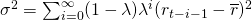where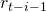is the daily return at t-i-1,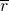is the average return, and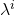is the smoothing factor. An alternative, more general way to present EWMA and which is perfectly equivalent, is the following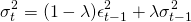where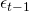is the (unexpected) return at t-1 and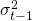is the volatility at t-1. Often, the recursion is initiated by using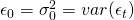. Lambda can be estimated, but is usually set at 0.94 for daily data.

## Volatility forecasting

The EWMA volatility measure provides an easy way to predict the volatility one day ahead. The advantage of this measure is that it does not require a lot of data and no complicated optimization methods are required.

## Summary

The exponentially weighted moving average volatility is a very simple measure of volatility that can be used to estimate volatility. It is often used in practice because it requires little data.

### EWMA volatility Excel

Want to implement the EWMA? Download the Excel file: EWMA Example.